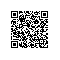# Application Center Test知识点滴积累

Application Center Test 在测试运行中自动监视 HTTP 性能统计信息，但性能计数器必须在测试运行之前明确进行配置。

DNS 错误

HTTP 错误

dcomcnfg.exe 启动 DCOM 配置工具

(sum of processor speed x processor use)
________________________________________  = cost, in cycles/request (or Hz/rps)
number of requests per second

((4 x 700 000 000 cycles/s) x 0.85)
___________________________________ = 2 975 000 cycles/request
800 requests/s

'''''''''''''''''''''''''''''''''''''''''''''''''''''''
' Function to return the cost of a page, based .
' stress test values and Web server/cluster hardware.
'
' Returns: page cost, in millions of cycles per request
'
Function GetPageCost(iSumOfProcessorSpeeds, _
fAverageProcessorUse, iAverageRPS)
Dim iSpentCyclesPerSecond
' calculate the number of cycles used over the testing period
iSpentCyclesPerSecond = iSumOfProcessorSpeeds _
* fAverageProcessorUse
' calculate the amount of work performed by the Web server
If (iAverageRPS > 0) Then
GetPageCost = iSpentCyclesPerSecond / iAverageRPS
Else
GetPageCost = 0
End If
End Function

page cost x target RPS
estimated processor speed = ______________________
processor use
2 975 000 cycles/request x 1600 requests/s
estimated processor speed = __________________________________________
0.40
estimated processor speed =  11 900 000 000 cycles/s
number of 700 MHz processors needed = 11 900 MHz / 700 MHz = 17使用钉钉扫一扫加入圈子
+ 订阅